## 1.排序问题

现有一个含有N个数字的数组S，如何通过程序把这个数组变成有序的数组？

例如：

排序前：S：5,3,7,5,9,4,1,100,50

排序后：S：1,3,4,5,5,7,9,50,100

## 2.二叉堆（binary heaps）

进行堆排序前，需要先把数组排成二叉堆，故这里先介绍二叉堆。

### 什么是二叉堆？

定义：二叉堆是一种特殊的堆，二叉堆是完全二元树（二叉树）或者是近似完全二元树（二叉树）。二叉堆有两种：最大堆和最小堆。最大堆：父结点的键值总是大于或等于任何一个子节点的键值；最小堆：父结点的键值总是小于或等于任何一个子节点的键值。

如果我们要用堆排序把数组排成递增有序的数组，则需要先排成最大堆；如果要把数组排成递减有序的数组，则需要先排成最小堆。

最大堆示意图：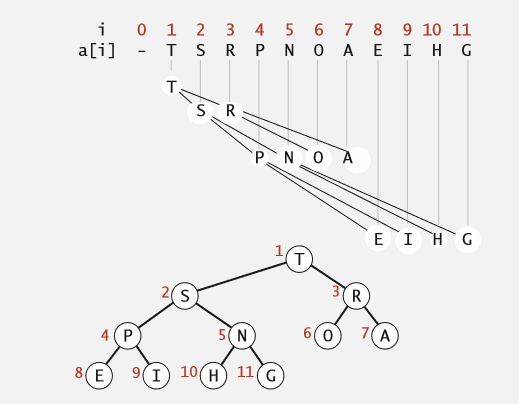上图为按照字母排序(如T>S>P）排成的最大堆。a和a为a的子节点，且a>=a, a>=a;a和a为a的子节点，且a>=a, a>=a。即父节点永远比子节点大(最小堆里，父节点永远比子节点小)。

即如果2k+1项元素在a[]数组中存在，则a[2k]和a[2k+1]为a[k]的子节点。

子节点间不一定是a[2k]>=a[2k+1]。

注意：最大堆的数组a[]是从a开始的！

### 如何把数组做出二叉堆？

这里介绍用从下而上的制作最大堆的方法：

令a[]数组是从a开始的N项数组。为方便解释，假设N=11，a[]数组如下图：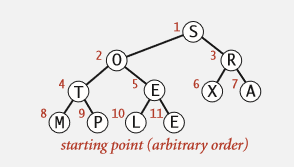先从int k = N/2（即k=5）开始讨论 ：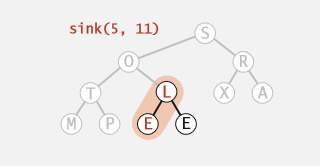1.首先确认int j = 2k; j+1项元素是否在数组中。在此例子中，j+1=11，j+1项元素在数组中。如果j+1项元素不存在，则直接跳到第3步。k项元素为父节点，j和j+1（如果有）项元素是子节点。

2.然后比较 j 项元素和 j+1 项元素大小：如果a[j+1] >= a[j] ， 则 j++;反之则不进行操作。这一步骤确保了 j 项元素是 k 项元素的最大的子节点。

3.然后比较 j 项元素和 k 项元素大小：如果 a[j] >= a[k] , 则交换 j 项元素和 k 项元素，将j的值赋给k, k = j；否则不进行操作。这一步让父节点和最大子节点进行比较，如果子节点大，则让父子节点交换，确保了父节点永远比子节点大。

4.检查k下面还有没节点：if(2 * k <= N)， 如果有，则继续 1,2,3,4步骤，直到下面没节点为止。这一步检查子节点是否有子节点，如果有，则确保子节点比它的子节点大。从而维持父节点永远比子节点大的结构特点。

在这里，k下面没节点了，检查k=4时的情况：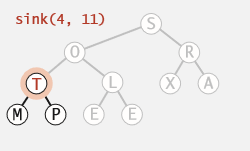执行1,2,3,4步骤后，检查k=3时的情况：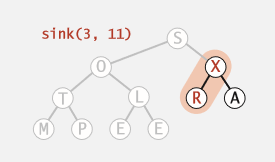执行1,2,3,4步骤后，检查k=2时的情况：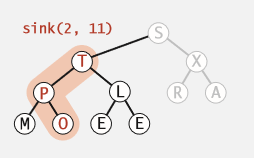执行1,2,3,4步骤后，检查k=1时的情况：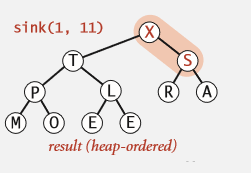这样，最大堆便形成了。

实现代码：(注意：heapsort是基于数组首项元素index为1的,而我们的数组首项元素index为0，因此，比较元素和交互元素时要减1。)

.h

public:
//下沉：针对一项元素，确保它大于等于子节点。
void Sink(int k, int N);
//开始排序
void Sort();
//i项元素比j项元素小？
bool Less(int i, int j);
//交换i项元素与j项元素
void Exchange(int i, int j);
private:
TArray<int> MyIntArray;

.cpp

void AMyHeapSort::Sort()
{
int N = MyIntArray.Num();
//排成最大堆
for (int k = N / 2; k >= 1; k--) Sink(k, N);
}

void AMyHeapSort::Sink(int k,int N)
{
//注意：heapsort是基于数组首项元素index为1的,而我们的数组首项元素index为0,故k-1项元素才是sink的目标元素；
//序号k应该在数组范围内
if (k > MyIntArray.Num()) return;
//2 * k是子元素的序号
while (2 * k <= N)
{
int j = 2 * k;
//如果隔壁的元素比较大，则用隔壁的
if (j < N && Less(j, j + 1)) j++;
//如果k项元素比子元素大，返回，下沉结束
if (!Less(k, j)) break;
//如果k项元素小于等于子元素，交换它们
Exchange(k, j);
//继续往下检查
k = j;
}
}

bool AMyHeapSort::Less(int i, int j)
{
//注意：heapsort是基于数组首项元素index为1的,而我们的数组首项元素index为0,故比较时减1；
//序号i,j应该在数组范围内
if (i > MyIntArray.Num() || j > MyIntArray.Num()) return false;
//比较大小
return MyIntArray[i-1] < MyIntArray[j-1];
}

void AMyHeapSort::Exchange(int i, int j)
{
//注意：heapsort是基于数组首项元素index为1的,而我们的数组首项元素index为0,故交换时减1；
//序号i,j应该在数组范围内
if (i > MyIntArray.Num() || j > MyIntArray.Num()) return;
MyIntArray.Swap(i - 1, j - 1);
}

## 3.堆排序（Heapsort）

数组形成最大堆后，只需简单几步就可以把完成堆排序：

1.把数组的第一个元素和最后一个元素交换，这样，数组中最大的元素就排到了数组的尾端。

2.把前N-1个元素看成一个数组。由于除了第一个元素，其它元素已经满足了最大堆的要求，所以对第一个元素执行Sink()，这个N-1个元素的数组就形成了最大堆。

3.重复1,2步骤，直到最后新形成的数组只有一个元素为止。

这样，数组就变成了有序递增的数组了，堆排序完成。

## 4.堆排序实现完整代码

.h

UCLASS()
class ALGORITHM_API AMyHeapSort : public AActor
{
GENERATED_BODY()

public:
// Sets default values for this actor's properties
AMyHeapSort();
// Called every frame
virtual void Tick(float DeltaTime) override;
//生成数组
void InitArray(int N);
//下沉：针对一项元素，确保它大于等于子节点。
void Sink(int k, int N);
//i项元素比j项元素小？
bool Less(int i, int j);
//交换i项元素与j项元素
void Exchange(int i, int j);
//开始排序
void Sort();
protected:
// Called when the game starts or when spawned
virtual void BeginPlay() override;

public:

private:
TArray<int> MyIntArray;

};

.cpp

// Sets default values
AMyHeapSort::AMyHeapSort()
{
// Set this actor to call Tick() every frame.  You can turn this off to improve performance if you don't need it.
PrimaryActorTick.bCanEverTick = true;

}

// Called when the game starts or when spawned
void AMyHeapSort::BeginPlay()
{
Super::BeginPlay();
//测试
//生成数组
InitArray(10000);
//排序前
for (int i = 0; i < MyIntArray.Num(); i++)
{
UKismetSystemLibrary::PrintString(this, "Before: " + FString::FromInt(i) + " : " + FString::FromInt(MyIntArray[i]));
}
Sort();
//排序后
for (int i = 0; i < MyIntArray.Num(); i++)
{
UKismetSystemLibrary::PrintString(this, "After: " + FString::FromInt(i) + " : " + FString::FromInt(MyIntArray[i]));
}
}

// Called every frame
void AMyHeapSort::Tick(float DeltaTime)
{
Super::Tick(DeltaTime);

}

void AMyHeapSort::InitArray(int N)
{
FRandomStream Stream;
Stream.GenerateNewSeed();
for (int i = 0; i < N; i++)
{
}
}

void AMyHeapSort::Sink(int k,int N)
{
//注意：heapsort是基于数组首项元素index为1的,而我们的数组首项元素index为0,故k-1项元素才是sink的目标元素；
//序号k应该在数组范围内
if (k > MyIntArray.Num()) return;
//2 * k是子元素的序号
while (2 * k <= N)
{
int j = 2 * k;
//如果隔壁的元素比较大，则用隔壁的
if (j < N && Less(j, j + 1)) j++;
//如果k项元素比子元素大，返回，下沉结束
if (!Less(k, j)) break;
//如果k项元素小于等于子元素，交换它们
Exchange(k, j);
//继续往下检查
k = j;
}
}

bool AMyHeapSort::Less(int i, int j)
{
//注意：heapsort是基于数组首项元素index为1的,而我们的数组首项元素index为0,故比较时减1；
//序号i,j应该在数组范围内
if (i > MyIntArray.Num() || j > MyIntArray.Num()) return false;
//比较大小
return MyIntArray[i-1] < MyIntArray[j-1];
}

void AMyHeapSort::Exchange(int i, int j)
{
//注意：heapsort是基于数组首项元素index为1的,而我们的数组首项元素index为0,故交换时减1；
//序号i,j应该在数组范围内
if (i > MyIntArray.Num() || j > MyIntArray.Num()) return;
MyIntArray.Swap(i - 1, j - 1);
}

void AMyHeapSort::Sort()
{
//注意：heapsort是基于数组首项元素index为1的,而我们的数组首项元素index为0,故k-1项元素才是sink的目标元素；
int N = MyIntArray.Num();
//排成最大堆
for (int k = N / 2; k >= 1; k--) Sink(k, N);
//不断地把最大的元素排在数组的尾端，形成有序递增数组
while (N > 1)
{
Exchange(1, N);
Sink(1,--N);
}
}